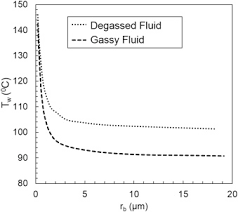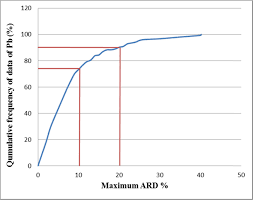## How to Calculate and Solve for Petrosky-Farshad Gas Solubility Parameter | The Calculator EncyclopediaThe image above represents petrosky-farshad gas solubility parameter.

To compute for the petrosky-farshad gas solubility parameter, two essential parameters are needed and these parameters are API Gravity (°API) and temperature (°Rankine) (T).

The formula for calculating the petrosky-farshad gas solubility parameter:

Rs = [7.716(10-4) (°API)1.541] – [4.561(10-5) (T -460)1.3911]

where:

Rs = Petrosky-Farshad Gas Solubility Parameter, x
°API = API Gravity
T = Temperature (°Rankine)

Let’s solve an example;
Find the petrosky-farshad gas solubility parameter with an API Gravity of 11 and a temperature of 90.

This implies that;

°API = API Gravity = 11
T = Temperature (°Rankine) = 90

Rs = [7.716(10-4) (°API)1.541] – [4.561(10-5) (T -460)1.3911]
Rs = [7.716(10-4) (11)1.541] – [4.561(10-5) (90 – 460)1.3911]
Rs = [7.716(10-4) (11)1.541] – [4.561(10-5) (-370)1.3911]
Rs = [7.716(10-4) (11)1.541] – [4.561(10-5) (NaN)]
Rs = [7.716(10-4) (40.2518)] – [4.561(10-5) (NaN)]
Rs = [0.03105] – [4.561(10-5) (NaN)]
Rs = 0.03105 – NaN
Rs = NaN

Therefore, the Petrosky-Farshad gas solubility parameter, x is NaN.

## How to Calculate and Solve for Petrosky-Farshad Gas Solubility in a Fluid | The Calculator EncyclopediaThe image above represents petrosky-farshad gas solubility.

To compute for the petrosky-farshad gas solubility, three essential parameters are needed and these parameters are gas specific gravity (γg), petrosky-farshad gas solubility parameter (x) and pressure (P).

The formula for calculating the petrosky-farshad gas solubility:

Rs = [(P/112.727 + 12.340)γg0.8439 10x]1.7384

Where:

γg = Gas Specific Gravity
x = Petrosky-Farshad Gas Solubility Parameter
P = Pressure

Let’s solve an example;
Find the petrosky-farshad gas solubility when the gas specific gravity is 16, the petrosky-farshad gas solubility parameter is 22 and the pressure is 80.

This implies that;

γg = Gas Specific Gravity = 16
x = Petrosky-Farshad Gas Solubility Parameter = 22
P = Pressure = 80

Rs = [(P/112.727 + 12.340)γg0.8439 10x]1.7384
Rs = [(80/112.727 + 12.340)160.8439 x 1022]1.9384
Rs = [(0.709 + 12.340)160.8439 x 1022]1.9384
Rs = [(13.049)160.8439 x 1022]1.9384
Rs = [(13.049)10.379 x 1022]1.9384
Rs = [1.354e+24]1.9384
Rs = 8.925e+41

Therefore, the Petrosky-Farshad gas solubility is 8.925e+41.